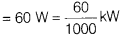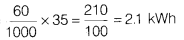# An electric bulb marked with 100 V-60 W is used for 5 h in a week. What is the cost of electricity, if the rate is ₹ 6 per unit?

An electric bulb marked with 100 V-60 W is used for 5 h in a week. What is the cost of electricity, if the rate is ₹ 6 per unit?

Given, power, PTime, t = 5 × 7 = 35h
Rate of electricity = ₹ 3 per unit
∴ The energy consumed in a week = Pt =The cost of electricity at the rate of = ₹ 3 per unit is = 3 × 2.1 = ₹ 6.3